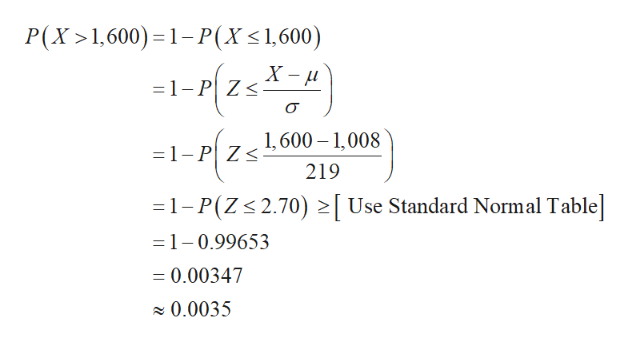# It is possible to score higher than 1600 on the combined mathematics and reading portions of the SAT, but scores 1600 and above are reported as 1600. Suppose the distribution of SAT scores (combining mathematics and reading) was approximately Normal with mean of 1008 and standard deviation of 219.What proportion of SAT scores for the combined portions were reported as 1600? That is, what proportion of SAT scores were actually higher than 1600? (Enter an answer rounded to four decimal places.)

Question
55 views

It is possible to score higher than 1600 on the combined mathematics and reading portions of the SAT, but scores 1600 and above are reported as 1600. Suppose the distribution of SAT scores (combining mathematics and reading) was approximately Normal with mean of 1008 and standard deviation of 219.

What proportion of SAT scores for the combined portions were reported as 1600? That is, what proportion of SAT scores were actually higher than 1600? (Enter an answer rounded to four decimal places.)

check_circle

Step 1

The proportion of SAT scores were actu...help_outlineImage TranscriptioncloseP(X 1,600) 1-P(X< 1,600) X- 1-P Z 1,600 1,008 1-P Z 219 1- P(Z 2.70) Use Standard Normal Table =1-0.99653 =0.00347 0.0035 fullscreen

### Want to see the full answer?

See Solution

#### Want to see this answer and more?

Solutions are written by subject experts who are available 24/7. Questions are typically answered within 1 hour.*

See Solution
*Response times may vary by subject and question.
Tagged in

### Other